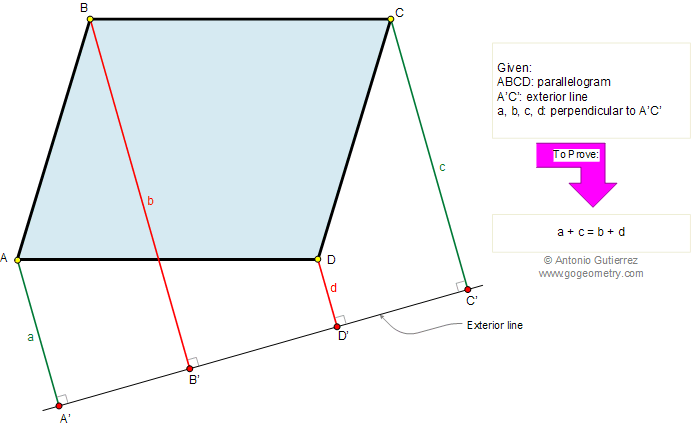Problem 233. Parallelogram, Exterior line, Perpendicular lines The figure shows a parallelogram ABCD, A'C' is an exterior line. If a, b, c, and d are the perpendiculars drawn from A, B, C, and D to the line A'C', respectively, prove that: a + c = b + d. View or post a solution.Home | Geometry | Problems | 231-240 | Parallelogram | Email | by Antonio Gutierrez